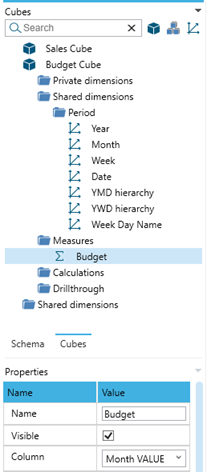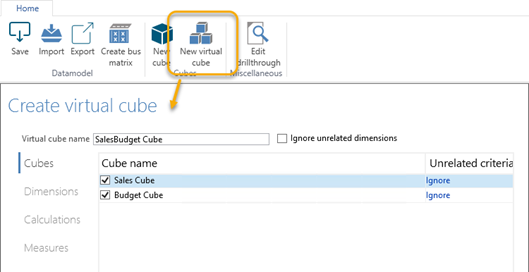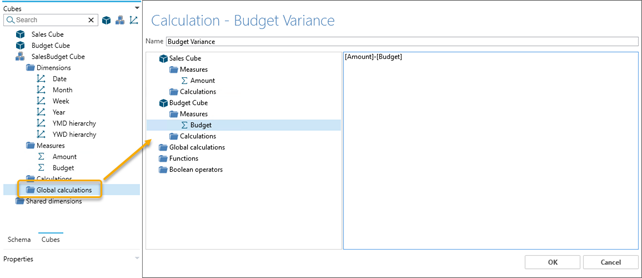# Virtual cubes

At this point we might want to add Budget measure (Month VALUE) to the existing Sales cube, but if we try, we will soon realize that it cannot be done.

The reality is that each cube in the Data Model Editor can only be designed from a single fact table, and the fact table for the Sales Cube is already defined as the Sales Invoice Line table.

Instead, we will need to create a new cube – with the Unpivot_BudgetData table as fact table – and subsequently create a Virtual Cube that combines relevant data from the other cubes.

First, create a Budget cube around the Unpivot_BudgetData table. Notice that the Budget measure is in fact the renamed Month VALUE from the Unpivot_BudgetData table.

Furthermore, the only relevant dimensions for the Budget data are the Period dimensions.Define the virtual cube by selecting relevant items from each of the included cubes:

• Cubes: Sales Cube and Budget Cube
• Dimensions: All dimensions related to Period
• Calculations: n/a
• Measures: Amount and Budget

Finally, we can create calculations upon the included measures in the virtual cube: